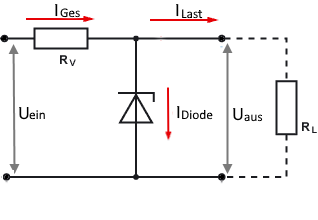# Zener diode as voltage regulator

Zener diode as voltage regulator calculator for circuits with a variable load current

### Calculate Series Resistor

This calculator calculates the series resistance of a Zener diode.

The resistance must be dimensioned that the total current does not pull down the output voltage.

A minimal current has to flow through the Zener diode for the stabilization to work. As a guide value, 10% of the load current or 10 mA can be assumed. A value of 10% of the maximum load current is automatically set; it can be overwritten if another value is preferred.

Zener diode resistor calculator

 Input Input voltage V Output voltage V Max. load current µA mA A Min. load current µA mA A Diode current µA mA A Decimal places 0 1 2 3 4 6 8 10 Results Series resistor Ser. resistor power Diode max. power Diode max. current Total current## Formulas and description

The total current of the circuit is determined by the series resistor and is always constant. When the load current decreases, the current through the Zener diode increases accordingly.

If the load current fluctuates between maximum and zero, the Zener diode must be dimensioned in such a way that that it can absorb the maximum current and power.

If a larger minimum current is specified, e.g. 300mA with a maximum current of 500mA, then the Zener diode only has to compensate for the difference of 200mA and can be dimensioned smaller.

However, the current must not fall below the minimum value. Otherwise the Zener diode can overheat and be destroyed.

 Total current $$\displaystyle I_{ges}=I_{Z\space min} + I_{L}$$ Diode current $$\displaystyle I_{D}= I_{L} · 0.1$$ Series resistor $$\displaystyle R_v=\frac{U_{ges} - U_Z}{I_{ges}}$$ Power of the series resistor $$\displaystyle P_v=(U_{ges} - U_Z) · I_{ges}$$ Power of the Diode $$\displaystyle P_D= U_Z · (I_{max} - I_{min} + I_D)$$